• A
• A
• A
• ABC
• ABC
• ABC
• А
• А
• А
• А
• А
Regular version of the site
Of all publications in the section: 25
Sort:
by name
by yearBook
Шакура Н. И., Постнов К. А., Липунова Г. В. и др. М.: Физматлит, 2016.BookBook
Алескеров Ф. Т., Андриевская И. К., Пеникас Г. И. и др. М.: Физматлит, 2013.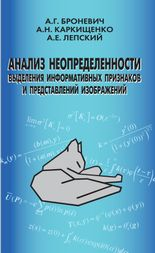Book
Броневич А. Г., Каркищенко А. Н., Лепский А. Е. М.: Физматлит, 2013.BookBookBook
Алескеров Ф.Т., Благовещенский Н., Сатаров Г. и др. М.: Физматлит, 2007.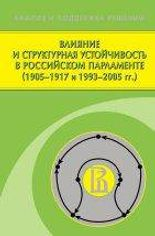Book
Алескеров Ф. Т., Благовещенский Н., Сатаров Г. А. и др. М.: Физматлит, 2009.Book
Панов А. И., Осипов Г. С., Чудова Н. В. и др. М.: Физматлит, 2017.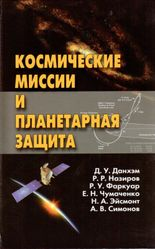Book
Данхэм Д. У., Назиров Р. Р., Фаркуар Р. и др. М.: Физматлит, 2013.Book
Коваленко Л., Власов В., Агаханов Н. и др. М.: Физматлит, 2002.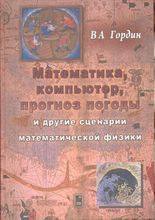Book
Гордин В. А. М.: Физматлит, 2013.Book
Осипов Г. С. М.: Физматлит, 2011.Book
Соколов А. В., Токарев В. В. Т. 1: Общие положения. Математическое программирование.. М.: Физматлит, 2012.

The manual is devoted to the mathematical theory and methods of optimization applied to  administrative decisions in economy. Volume 1 described approaches to mathematical modeling of management problems in economy and methods of mathematical programming tasks solution. Besides strict mathematical proofs, there are directing reasons, which is sometimes enough for understanding. There are many economic examples and exercises with detailed solutions. Readers are supposed to know the bases of the mathematical analysis and linear algebra, though necessary data from these courses in a concise form are provided in appendices.Book
Токарев В. В. М.: Физматлит, 2010.Book
Токарев В. В., Соколов А. В. Т. 1. М.: Физматлит, 2011.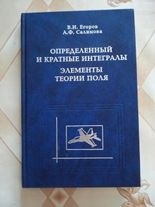Book
Егоров В. И., Салимова А. Ф. М.: Физматлит, 2004.